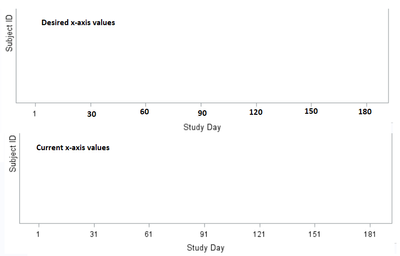## proc sgplot - how to show / force fixed values on x-axis?

Hi Folks,

In my swimmer's plot, I have to show Day 1 on x-axis, however, specifying 1 to 210 by 30 make the following measurement points 31, 61, 91, 121 and 181. How to force Day 1 while the rest of the time points on x-axis show 30, 60, 90 and 180 instead?

``````PROC SGPLOT DATA=SWIMMER;
HIGHLOW Y=ORDER LOW=TRTSTDY HIGH=TRTENDY / LOWLABEL=SUBJID TYPE=BAR;
XAXIS LABEL='Study Day' VALUES=(1 TO 210 BY 30);
RUN;
``````1 ACCEPTED SOLUTION

Accepted Solutions

## Re: proc sgplot - how to show / force fixed values on x-axis?

Which is exactly what you told it to do with "by 30" that adds 30 to each value (and since the 181+30 is greater than 210 does not display the calculated 211)

Try :

values = (1, 30 to 210 by 30)

## Re: proc sgplot - how to show / force fixed values on x-axis?

Which is exactly what you told it to do with "by 30" that adds 30 to each value (and since the 181+30 is greater than 210 does not display the calculated 211)

Try :

values = (1, 30 to 210 by 30)

Discussion stats